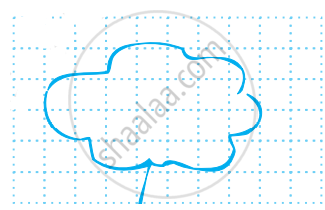# By counting squares, estimate the area of the figure. - Mathematics

Sum

By counting squares, estimate the area of the figure.#### Solution

 Covered area Number Area estimate (sq units) (i) Fully-filled squares 11 11 (ii) Half-filled squares 3 3 xx 1/2 (iii) More than half-filled squares 7 7 (iv) Less than half-filled squares 5 0

Total area = 11 + 3 × 1/2 + 7 = 19 1/2 sq units.

Concept: Concept of Area
Is there an error in this question or solution?

Share